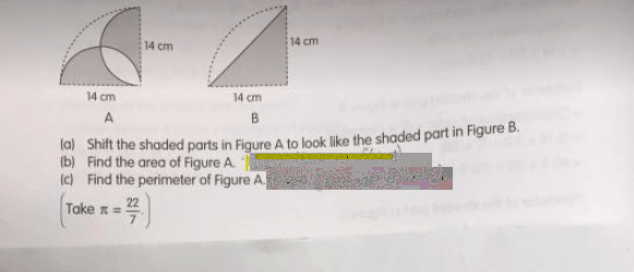# Question(a)
In Figure A, draw a line from the top left corner from Figure A to its bottom left which will look like the one in Figure B. Shift the two segments to fill the overlapping semicircles tyo obtain the shaded triangle in Figure B.

(b)
shaded area of Figure A = shaded area of Figure B = (1/2) x 14 x 14 = 98
or
(1/4) x (22/7) x 7 x 7 = 38.5
(1/2) x 7 x 7 = 24.5
(1/2) x (22/7) x 7 x 7 + [(7 x 7) – (1/4) x (22/7) x 7 x 7] x 2 = 98

(c)
perimeter of figure A = (22/7) x 14 + 2 x 14 = 72

Ans : (a) as above; (b) 98 sq cm; (c) 72 cm.

0 Replies 0 Likes